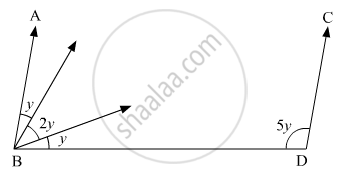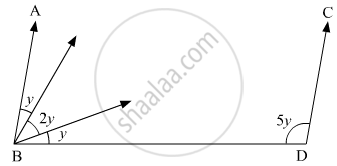# In the Given Figure, If Line Segment Ab is Parallel to the Line Segment Cd, What is the Value of Y? - Mathematics

MCQ

In the given figure, If line segment AB is parallel to the line segment CD, what is the value of y?• 12

• 15

• 18

• 20

#### Solution

The figure is given as follows:It is given that AB is parallel to CD.

Thus,∠ABDand

$\angle BDC$ are consecutive interior angles.

Therefore, their sum must be supplementary.

That is,

$\angle ABD + \angle BDC = 180^\circ$

From the figure, we get:

( y + 2y + y ) + (5y) = 180°

4y+ 5y = 180°

9y = 180°

 y =(180°)/9

y = 20°

Concept: Parallel Lines and a Transversal
Is there an error in this question or solution?
Chapter 10: Lines and Angles - Exercise 10.6 [Page 57]

#### APPEARS IN

RD Sharma Mathematics for Class 9
Chapter 10 Lines and Angles
Exercise 10.6 | Q 29 | Page 57

Share